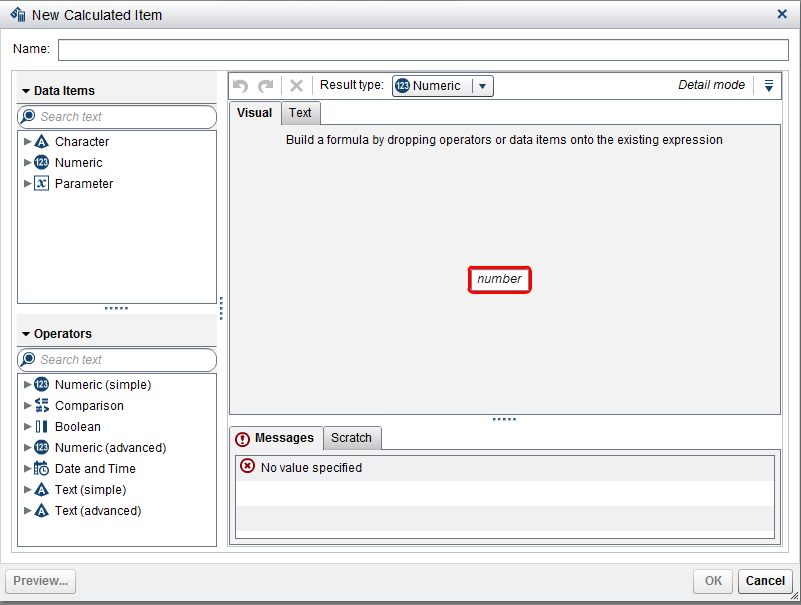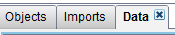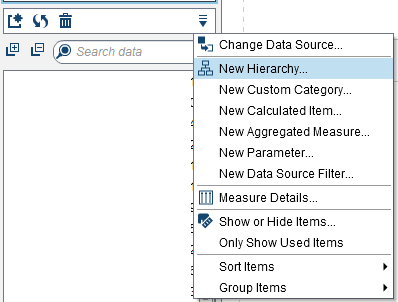## Adding percentages to Cross Tabs in SAS VA

Hi

Is there a way to add row percentages in a cross tab or even a list table in SAS VA?

For example,  I have 3 columns, A,B,C and want to then get a total column of A+B+C.

I then want to have a column that gives me the percentage of A out of the total column.

Also, is it possible  to do column A / column B to give me that precentage?

In Excel it would be  '=A1/B1' in the new column to give me the percentage. Is this doable in SAS VA?

1 ACCEPTED SOLUTION

Accepted Solutions

## Re: Adding percentages to Cross Tabs in SAS VA

Yes this is possible. You need to add a new calculated item.You can find the option on the tab 'Data'Select option new calculated item

2 REPLIES 2

## Re: Adding percentages to Cross Tabs in SAS VA

Yes this is possible. You need to add a new calculated item.You can find the option on the tab 'Data'Select option new calculated item

## Re: Adding percentages to Cross Tabs in SAS VA

Hi

That worked thanks.

When the calculated item has been put into the list or crosstab I found that you needed to select  'average' as the aggregation for it to do the correct calculation.

When I created it as a new aggregated measure instead then it automatically set it as the correct calculation.

I had to remember to change the format to percentage too.

Thanks

Discussion stats
• 2 replies
• 1944 views
• 0 likes
• 2 in conversation